• Python 大作业 xx 游戏 姓名 学号 姓名 学号 一游戏介绍 我们设计的是五子棋游戏支持两人一个鼠标对下黑方用左键单击白方用右键单击谁先下均可落子无悔下过的棋子对方点击后不会变色程序可自行判断输赢并在五子连珠...
• Python五子棋程序设计课程大作业五子棋游戏，支持两人一个鼠标对下，黑方用黑子代替，白方用白子代替，谁先下均可，落子无悔，下过的棋子对方点击后也不可更改。游戏双方需遵循一次下一子的规则。
• 是上Python课程的期末大作业，设计五子棋游戏程序，界面都有
• python大作业大全游戏五子棋、贪吃蛇、拼图、坦克大战）
• Python大作业源码五子棋AI 坦克大战AI贪吃蛇滑雪游戏拼图7个小游戏合集: FlappyBird Python实现AI贪吃蛇 五子棋AI 强火车票下 拼图 简单的滑雪游戏 经典90坦克大战
• 临近期末考试，班主任把所有的不参与考试的副科课程全都停了，天天语数外历史物理，实在是无聊，同学们便在课间互相约战五子棋，棋盘便是平时写作业用的玛丽大号写字本，棋子就是xo，不到两个星期，我就成功地用完了...
临近期末考试，班主任把所有的不参与考试的副科课程全都停了，天天语数外历史物理，实在是无聊，同学们便在课间互相约战五子棋，棋盘便是平时写作业用的玛丽大号写字本，棋子就是xo，不到两个星期，我就成功地用完了一本32页的写字本（心疼我的写字本三秒钟），这也太浪费纸了吧！教室里都装有触屏的电子白板，又为什么不让他们在电脑上下呢？而且还是全班直播，多好！
出于这个念头，我便用Python的pygame库开发了一个单机双人的五子棋小游戏，以供大家娱乐。
Talk is cheap,show me the code.（话不多说，上代码）：
import pygame
import time
import sys
import easygui
from pygame.locals import *

initChessList = []
initRole = 1
resultFlag = 0

class StornPoint():
def __init__(self, x, y, value):
'''
:param x: 代表x轴坐标
:param y: 代表y轴坐标
:param value: 当前坐标点的棋子：0:没有棋子 1:白子 2:黑子
'''
self.x = x
self.y = y
self.value = value

def initChessSquare(x, y):
for i in range(15):
rowlist = []
for j in range(15):
pointX = x + j*40
pointY = y + i*40
sp = StornPoint(pointX, pointY, 0)
rowlist.append(sp)
initChessList.append(rowlist)

def eventHander():
for event in pygame.event.get():
global initRole
if event.type == QUIT:
pygame.quit()
sys.exit()
if event.type == MOUSEBUTTONDOWN:
x, y = pygame.mouse.get_pos()
i = 0
j = 0
for temp in initChessList:
for point in temp:
if x >= point.x-10 and x <= point.x+10 and y >= point.y-10 and y <= point.y+10:
if point.value == 0 and initRole == 1:
point.value = 1
judgeResult(i, j, 1)
initRole = 2
elif point.value == 0 and initRole == 2:
point.value = 2
judgeResult(i, j, 2)
initRole = 1
break
j += 1
i += 1
j = 0

def judgeResult(i, j, value):
global resultFlag
flag = False
for x in range(j - 4, j + 5):
if x >= 0 and x + 4 < 15:
if initChessList[i][x].value == value and \
initChessList[i][x + 1].value == value and \
initChessList[i][x + 2].value == value and \
initChessList[i][x + 3].value == value and \
initChessList[i][x + 4].value == value:
flag = True
break
pass
for x in range(i - 4, i + 5):
if x >= 0 and x + 4 < 15:
if initChessList[x][j].value == value and \
initChessList[x + 1][j].value == value and \
initChessList[x + 2][j].value == value and \
initChessList[x + 3][j].value == value and \
initChessList[x + 4][j].value == value:
flag = True
break
pass

for x, y in zip(range(j + 4, j - 5, -1), range(i - 4, i + 5)):
if x >= 0 and x + 4 < 15 and y + 4 >= 0 and y < 15:
if initChessList[y][x].value == value and \
initChessList[y - 1][x + 1].value == value and \
initChessList[y - 2][x + 2].value == value and \
initChessList[y - 3][x + 3].value == value and \
initChessList[y - 4][x + 4].value == value:
flag = True

for x, y in zip(range(j - 4, j + 5), range(i - 4, i + 5)):
if x >= 0 and x + 4 < 15 and y >= 0 and y + 4 < 15:
if initChessList[y][x].value == value and \
initChessList[y + 1][x + 1].value == value and \
initChessList[y + 2][x + 2].value == value and \
initChessList[y + 3][x + 3].value == value and \
initChessList[y + 4][x + 4].value == value:
flag = True

if flag:
resultFlag = value
print("白棋胜" if value == 1 else "黑棋胜")
easygui.msgbox("白棋胜" if value == 1 else "黑棋胜")

def main():
global initChessList, resultFlag
initChessSquare(27, 27)
pygame.init()
screen = pygame.display.set_mode((620, 620), 0, 0)
pygame.display.set_caption("五子棋")
background = pygame.image.load("images/bg.png")
whiteStorn = pygame.image.load("images/storn_white.png")
blackStorn = pygame.image.load("images/storn_black.png")
rect = blackStorn.get_rect()

while True:
screen.blit(background, (0, 0))
for temp in initChessList:
for point in temp:
if point.value == 1:
screen.blit(whiteStorn, (point.x-18, point.y-18))
elif point.value == 2:
screen.blit(blackStorn, (point.x-18, point.y-18))

if resultFlag > 0:
initChessList = []
initChessSquare(27, 27)
pygame.display.update()

if resultFlag > 0:
time.sleep(3)
resultFlag = 0
eventHander()

if __name__ == '__main__':
main()
pass


下面是程序中用到的图片素材 ：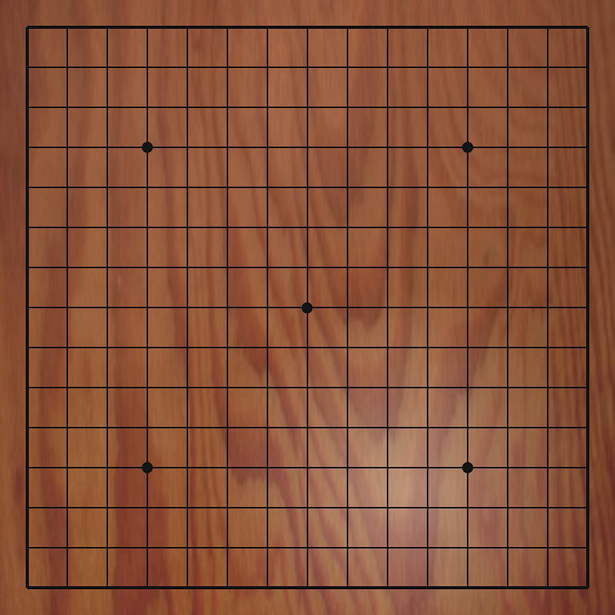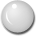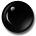展开全文pygame
• python大作业分享--30多个项目任你选（由于上传大小限制分多个文件上传。爬虫：https://download.csdn.net/download/weixin_43960044/12533382小游戏：https://download.csdn.net/download/weixin_43960044/12533379...
• python大作业实验报告+源代码--爬虫【完美搞定大作业python大作业分享--30多个项目任你选（由于上传大小限制分多个文件上传。爬虫https://download.csdn.net/download/dhyuan_88/19651195小游戏：...
• 请设计一个简单的五子棋游戏，棋盘大小为15*15，黑子先落。可以右击鼠标来悔棋，可以无限制悔棋直到棋盘无棋子。在每次下棋子之前，先判断该处有无棋子，有则不能落子，超出边界不能落子。任何一方有达到横向、竖向...
Python小作业
图片资源，放在和代码同级目录即可，注意文件名
请自行安装依赖的库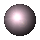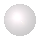五子棋是一种家喻户晓的休闲益智游戏，它最大的优点在于游戏规则简单、上手快、趣味性强。请设计一个简单的五子棋游戏，棋盘大小为15*15，黑子先落。可以右击鼠标来悔棋，可以无限制悔棋直到棋盘无棋子。在每次下棋子之前，先判断该处有无棋子，有则不能落子，超出边界不能落子。任何一方有达到横向、竖向、斜向或反斜向连到5个棋子则胜利，弹出胜利提示窗口。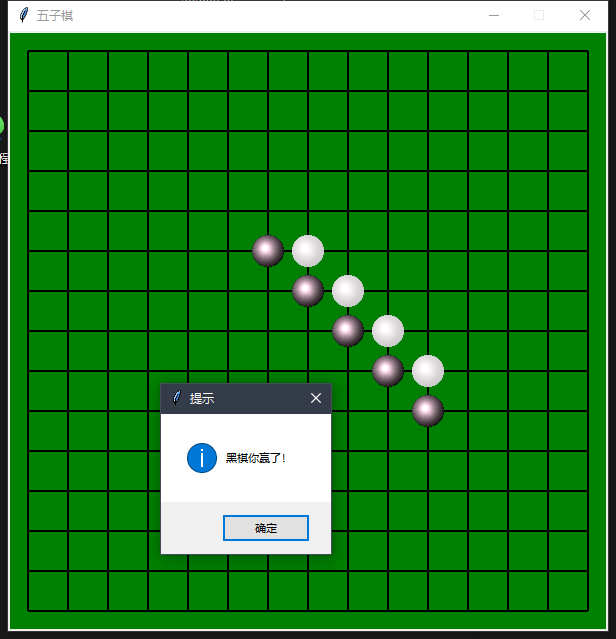# @data   2020-05-11 19:56

from tkinter import *
from PIL import Image, ImageTk
import tkinter.messagebox

class Chess:
@staticmethod
def center(window, w, h):  # 设置窗口大小且居中
ws = window.winfo_screenwidth()
hs = window.winfo_screenheight()
x = (ws / 2) - (w / 2)
y = (hs / 2) - (h / 2)
window.geometry("{:.0f}x{:.0f}+{:.0f}+{:.0f}".format(w, h, x, y))

def is_win(self):
for i, j in self.seq_list:
color = self.matrix_flag[i][j]
if j <= 10:
for col in range(j + 1, j + 5):  # 判断横向
if self.matrix_flag[i][col] != color:
break
else:
tkinter.messagebox.showinfo("提示", "黑棋你赢了！" if color == 1 else "白棋你赢了！")
return
if i <= 10:
for row in range(i + 1, i + 5):  # 判断纵向
if self.matrix_flag[row][j] != color:
break
else:
tkinter.messagebox.showinfo("提示", "黑棋你赢了！" if color == 1 else "白棋你赢了！")
return

if i <= 10 and j <= 10:
for row, col in zip([x for x in range(i + 1, i + 5)], [y for y in range(j + 1, j + 5)]):  # 对角线判断
if self.matrix_flag[row][col] != color:
break
else:
tkinter.messagebox.showinfo("提示", "黑棋你赢了！" if color == 1 else "白棋你赢了！")
return

def undo(self, event):  # 悔棋
if self.seq_list:
i, j = self.seq_list.pop()
self.canvas.delete(self.matrix_img[i][j])
self.matrix_img[i][j] = None
self.matrix_flag[i][j] = 0
else:
tkinter.messagebox.showwarning("警告", "已经没有任何棋子了！")

def callback(self, event):  # 落子
x, y = event.x - 20, event.y - 20
res_x, res_y = x // 40, y // 40
div_x, div_y = x % 40, y % 40
flag_x = flag_y = False  # 分布判断 x , y的坐标是否在交点周围
i = j = 0
if div_x <= 10:
flag_x = True
x = res_x * 40
j = res_x
elif 30 <= div_x:
flag_x = True
x = (res_x + 1) * 40
j = res_x + 1
if div_y <= 10:
flag_y = True
y = res_y * 40
i = res_y
elif 30 <= div_y:
flag_y = True
y = (res_y + 1) * 40
i = res_y + 1

if flag_x and flag_y and not self.matrix_flag[i][j]:
self.matrix_img[i][j] = self.canvas.create_image(x + 20, y + 20, image=self.img_black)
self.img_black, self.img_white = self.img_white, self.img_black  # 黑白子交换
self.matrix_flag[i][j] = self.count % 2 + 1  # 黑子为 1 白子为 2
self.count += 1
self.seq_list.append((i, j))
self.is_win()  # 判断是否达到五个

def __init__(self):
self.row, self.column = 15, 15
self.matrix_flag = [[0 for _ in range(self.row)] for _ in range(self.column)]
self.matrix_img = [[None for _ in range(self.row)] for _ in range(self.column)]
self.count = 0
self.seq_list = []
self.root = Tk()
self.root.resizable(width=False, height=False)  # 设置窗口不可缩放
self.root.title("五子棋")
self.center(self.root, 600, 600)
self.canvas = Canvas(self.root, bg="green", bd=0)
self.canvas.pack(fill='both', expand='YES')
self.img_black = ImageTk.PhotoImage(Image.open("blackstone.gif"))  # 加载黑棋
self.img_white = ImageTk.PhotoImage(Image.open("whitestone.gif"))  # 加载白棋
self.draw_grid()

self.canvas.bind("<Button-1>", self.callback)  # 落子
self.canvas.bind("<Button-3>", self.undo)  # 悔棋

self.root.mainloop()

def draw_grid(self):  # 绘制网格
start = [(20, i) for i in range(20, 580, 40)] + [(i, 20) for i in range(20, 580, 40)] + [(20, 580), (580, 20)]
end = [(580, i) for i in range(20, 580, 40)] + [(i, 580) for i in range(20, 580, 40)] + [(580, 580), (580, 580)]
for i in range(len(start)):
self.canvas.create_line((start[i], end[i]), width=2)

if __name__ == '__main__':
Chess()



展开全文canvas tkinter
• 最近刚好有空，整理一下上学期《人工智能基础》课程的大作业项目。 四个实验代码已上传至我的github：https://github.com/TommyGong08/Gobang_AI 有兴趣的小伙伴可以star 欢迎关注我的github，这对我来说很重要~ ...
人工智能：五子棋项目
系列文章目录
【章节总结】人工智能基础——搜索与问题求解
【课程项目】BIT人工智能大作业：五子棋游戏

这是我的第一篇博客。
最近刚好有空，整理一下上学期《人工智能基础》课程的大作业项目。四个实验代码已上传至我的github：https://github.com/TommyGong08/Gobang_AI
有兴趣的小伙伴可以star 也欢迎关注我的github~

问题一——棋子识别
方法
监督学习算法——采用YOLO-tiny（基于keras）网上开源代码很多
环境依赖

python3.6
NumPy
SciPy
matplotlib
keras==2.1.5
tensorflow==1.6.0
tensorflow-gpu==1.8.0

效果
个人感觉勉强可以，毕竟数据集不是自己做的，来自大佬@Sharpiless
棋子不密集棋子比较密集棋子很密集显然，棋子密集处检测效果比较不佳
问题二——搜索算法
采用alpha-beta剪枝对棋局局面进行搜索
设计评估方法
具体评估方法如下所示：

遍历棋盘上的每一个点，如果存在棋子，则对这个点所在的四个方向形成的四条线分别进行评估。
对于具体的一条线，如下图，已选取点为中心，取该方向上前面四个点，后面四个点，组成一个长度为9的数组。

评分规则
必杀情况的评分能够直接得出，评分规则如表2-1所示，最高的分为连五局面下的10000分的，最低分为-10000分；当黑棋出现连四，评分为9050分，当白棋出现连四，评分为-9050分；除了表中情况外，其余情况下针对黑棋或白棋的活三，眠三，活二，眠二的个数依次增加分数，评分为（黑棋得分 – 白棋得分）。效果
设置搜索深度为2，进行一场五子棋对局，五子棋AI取得胜利，搜索算法和评估函数有效提升棋力。搜索深度与计算时间
由于极大极小值的本质是深度优先搜索，通过调整搜索深度，可以得到搜索时间与搜索深度之间的关系。依次设置搜索深度为1，2，3，4，可以测得平均搜索时间分别为0.02s, 0.21s, 2.50s, 30.0s。根据所测得数据做出如下搜索深度与搜索时间关系图如下。问题三——基于ANN的棋局评估
思路
将实验二中的棋局判断函数更改为人工神经网络模型，并采用进化计算对该网络模型进行学习，达到提升五子棋博弈水平的目的。
方法

基于Keras和Tensorflow深度学习框架搭建神经网络
在第二问的基础上改进方案，让黑白两色AI对局200次，目的是生成训练所用的数据集。
得到数据集，采用进化计算的方法训练人工神经网络。
将第二问中的评估函数替换成我们所训练的神经网络，让神经网络拟合棋局评估值。

构建神经网络
基于Keras和Tensorflow框架搭建神经网络，网络为全连接结构，含有一个输入层，四个隐含层，一个输出层，输入层有32个神经元，隐含层分别有64，128，64，8个神经元，输出层含有1个神经元。损失函数为MSE,优化器为adam。数据集格式
在前两个实验的过程中，我所涉及的棋盘大小均为1919。因此，在本实验中记录两个数据集，分别为x_train和y_train，两者均为文本文件。x_train的每一行为1361大小的数组，因为19*19等于361。
训练过程

调整迭代次数epochs
首先将epochs设置为1000次，因为1000为较大的数字，所以从1000逐渐递减，发现epochs在200至1000之间loss差距不大。
因此确定epochs为200最佳。
调整batch_size
初始化batch_size为15，随后调整batch_size从15递减至2，发现损失函数均收敛至0.17附近。本实验选取epochs为200和batch_size为10的组合

结果
训练完成模型后，编写函数接口调用网络模型，对于每一个局面计算评估函数。与此时的AI对局一次，可以发现棋子AI落子合理，最终真人玩家取得胜利。下面两张图展示了epochs为200时，batch_size分别为12和10时的损失函数图，训练过程中，损失函数呈现下降趋势。其中，通过左图可以观察到batch_size等于12时，loss先将过程中震荡更大，然而并不影响最终loss均收敛之0.17左右。问题四——基于DQN的棋力提升
思路
采用强化学习算法对实验三的人工神经网络模型进行学习，使得五子棋博弈程序的下棋水平不断提高。
训练流程

构造卷积神经网络估计V值
让黑色与白色双方互博积累经验
记录胜利的局面，并加入到数据集中
V值网络每记录100个数据进行一次训练

效果

强化学习前
AI落子方式为随机落子，如图5-1所示，可以观察到棋局混乱，杂乱无章。2. 强化学习后
训练次数达到20此后，可以观察到AI落子下棋能力得到增强，下棋更有章法。感兴趣的小伙伴可以欢迎收藏~


展开全文神经网络 tensorflow
• python大作业分享--30多个项目任你选（由于上传大小限制分多个文件上传。爬虫：https://download.csdn.net/download/weixin_43960044/12533382小游戏：https://download.csdn.net/download/weixin_43960044/12533379...
• python大作业实验报告+源代码--爬虫【完美搞定大作业python大作业分享--30多个项目任你选（由于上传大小限制分多个文件上传。爬虫https://download.csdn.net/download/dhyuan_88/19651195小游戏：...
• python三子棋（又叫九宫格，一条龙等）游戏大作业。某学校的python课程大作业，当年帮助别人写的，比五子棋简单一些。需要用到graphics.py库（这里免积分下载：...
• python大作业有哪些项目 选题做个啥 python大作业分享--30多个项目任你选（由于上传大小限制分多个文件上传。爬虫https://download.csdn.net/download/dhyuan_88/19651195小游戏：...
python大作业有哪些项目 选题做个啥【推荐】

python大作业分享--30多个项目任你选（由于上传大小限制分多个文件上传。

爬虫：https://download.csdn.net/download/dhyuan_88/19651195

小游戏：https://download.csdn.net/download/dhyuan_88/19651189

python大作业有哪些项目 选题做个啥？应付大作业完全没问题。内涵30多个项目，随意挑选。

爬虫（爬抖音视频、下载B站视频、怕天气预报等）小游戏（五子棋、坦克大战、贪吃蛇、拼图等）。

展开全文• python学习(游戏、爬虫、排序、练习题、错误总结) ...l7bz python100经典练习题 ...python-大作业五子棋游戏（附代码） python-网络爬虫 几种排序方法python实现 新手常见的python运行时错误汇总 ...
• 原标题：拯救Python新手的几个项目实战Python 做小游戏实例一：24点游戏项目名称：经典趣味24点游戏程序设计(python) 实例二：五子棋游戏python学习关注我们企鹅qun： 8393 83765 各类入门学习资料免费分享哦！...
• 原标题：拯救Python新手的几个项目实战Python 做小游戏实例一：24点游戏项目名称：经典趣味24点游戏程序设计(python) 实例二：五子棋游戏python学习关注我们企鹅qun： 8393 83765 各类入门学习资料免费分享哦！...
• 原标题：拯救Python新手的几个项目实战Python 做小游戏实例一：24点游戏项目名称：经典趣味24点游戏程序设计(python) 实例二：五子棋游戏python学习关注我们企鹅qun： 8393 83765 各类入门学习资料免费分享哦！...
• Python 做小游戏 ...项目名称：python大作业 五子棋 人人对战 实例三：2048小游戏 项目名称：Python实例浅谈之八2048游戏（字符界面） 实例四：街机小游戏 项目名称：DIY街机游戏 实例五：扫雷小...
• canvas 游戏 (这个搞定好多好玩的idea可以实现了php 暂时不想玩各种框架吧,用原生php做一个小型的cmspython 唔,bzoj貌似wget爬不到,目前想直接用python强行爬java 唔,这学期java课不上的代价就是做个大作业,我找了...# python五子棋游戏大作业python 订阅